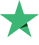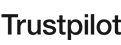watching now
1 Students
43 Lessons
Intermediate

#### What Will You Learn?

• Derivatives and their geometrical interpretation
• Definite and indefinite integrals
• Limits and continuity
• Usage of parabolas, ellipses, and hyperbolas
• and so much more!

#### Curriculum

4h
02:28
##### Section 2: Derivatives 1
37:19
Derivatives Example 5
06:43
Derivatives Example 6
01:22
##### Section 3: Derivatives 2
30:31
Derivatives Example 1
13:33
Derivatives Example 2
03:31
Derivatives Example 3
03:31
Derivatives Example 4
02:35
Derivatives Example 5
02:30
Derivatives Example 6
04:51
##### Section 4: Derivatives 3
28:07
Derivatives Example 1
08:29
Derivatives Example 2
03:26
Derivatives Example 4
02:58
Derivatives Example 5
11:29
Derivatives Example 6
01:45
##### Section 5: Derivatives 4
25:56
Derivatives Example 1
05:36
Derivatives Example 2
03:13
Derivatives Example 3
03:12
Derivatives Example 4
02:52
Derivatives Example 5
06:35
Derivatives Example 6
04:28
##### Section 6: Derivatives 5
27:23
Derivatives Example 1
03:49
Derivatives Example 2
02:29
Derivatives Example 3
03:15
Derivatives Example 4
02:48
Derivatives Example 5
06:57
Derivatives Example 6
05:36
Derivatives Example 7
02:29
##### Section 7: Derivatives 6
45:39
Derivatives Example 1
09:51
Derivatives Example 2
05:43
Derivatives Example 3
02:52
Derivatives Example 4
06:16
Derivatives Example 5
02:24
Derivatives Example 6
18:33
##### Section 8: Derivatives Example 7
43:18
Derivatives Example 1
08:29
Derivatives Example 2
06:48
Derivatives Example 3
12:59
Derivatives Example 4
05:34
Derivatives Example 5
01:53
Derivatives Example 6
07:35

#### Requirements

• Basics in algebra
• Basics in geometry
• A pen and a notebook

Reviews 2
Students 19
Courses 3

If there is a walking encyclopedia of Calculus and solving differential equations, then it should be called Ad Chauhdry.

Ad Chauhdry is a researcher of mathematics for over 15 years in which he’s contributed with articles in several scientific journals with good impact factor. His work also includes teaching post-graduate students, so you can trust his teaching and researching experience. Apart from working in an academic environment, Ad Chauhdry has also established an online and offline academy called AD Maths Plus Academy. 20k+ students have enrolled in AD Maths Plus courses.

With Ad Chauhdry, you may jump into learning how to solve differential equations and then transfer your skills into learning Python on BitDegree. He’s also created a course for you to get a grasp of Calculus / Analytic geometry, so you can build a solid foundation for further learning of tech skills. Ad Chauhdry reveals the secrets of maths and differential equations underlying so many things in economics, technology development, etc. If you want to see it for yourself, you have an excellent opportunity to get such things explained by an experienced mathematician, scientist, researcher, and educator – Ad.

Ad and his wife (also a teacher) are growing 5 children – this should prove to you that he’s probably quite good at assisting younger generations. Join Ad’s courses and dive straight into learning!

#### BitDegree platform reviews

Our students say Excellent
9.5 out of 10